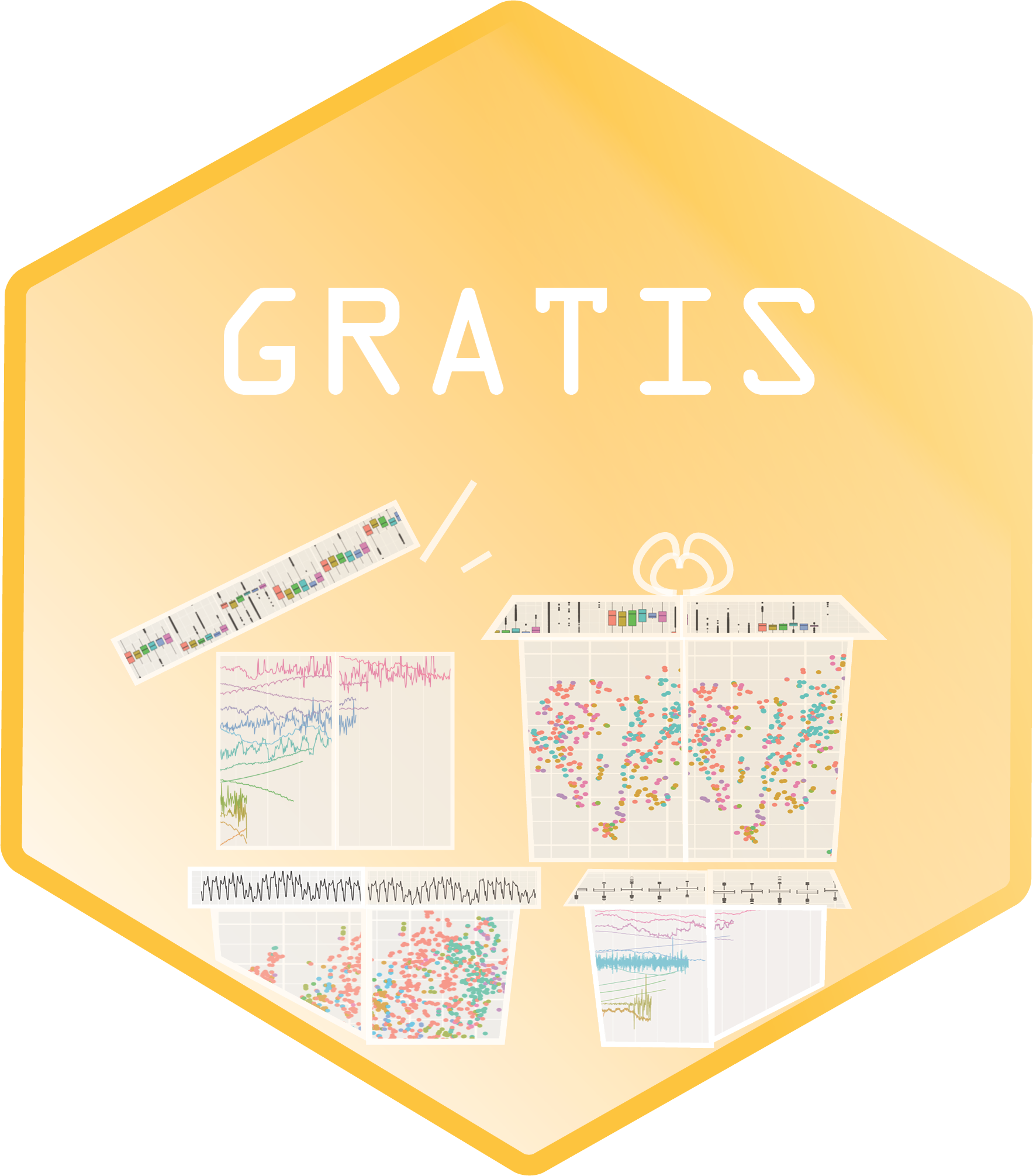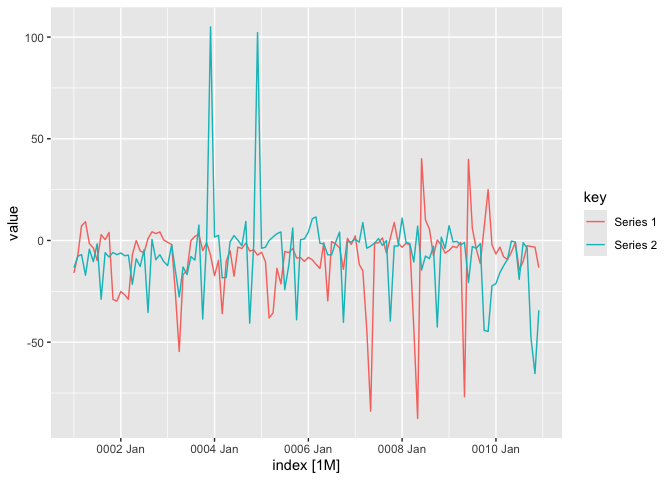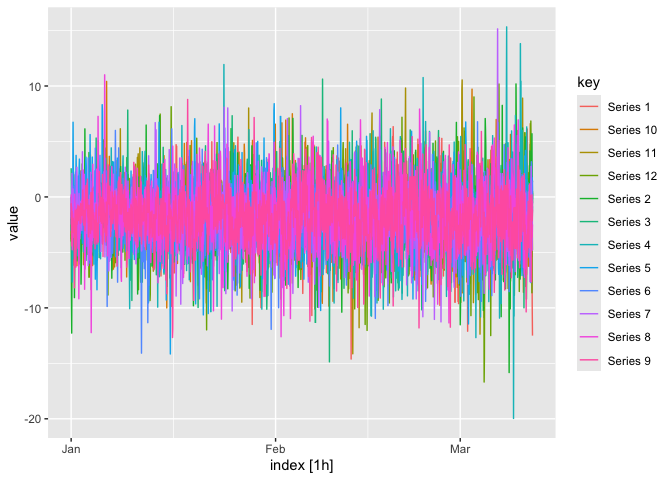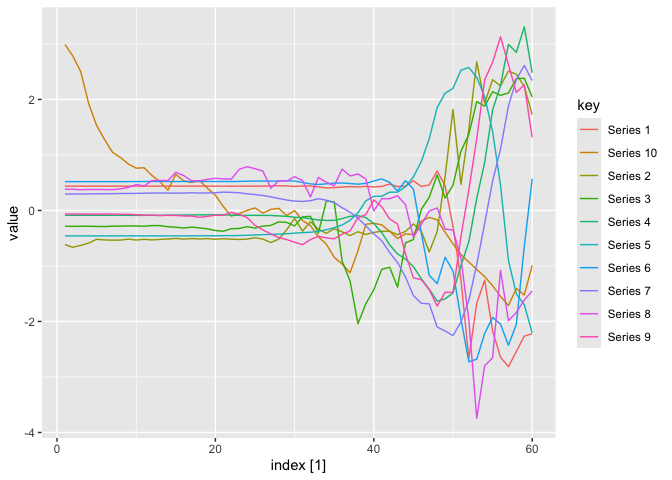# gratisThe R package `gratis` (previously known as `tsgeneration`) provides efficient algorithms for generating time series with diverse and controllable characteristics.

## Installation

### CRAN version

``install.packages("gratis")``

### Development version

You can install the development version of `gratis` package from GitHub Repository with:

``devtools::install_github("ykang/gratis")``

## Usage

### Tutorial video

Watch this YouTube video provided by Prof. Rob Hyndman.

``````library(gratis)
library(feasts)``````

### Generate diverse time series

``````set.seed(1)
mar_model(seasonal_periods=12) %>%
generate(length=120, nseries=2) %>%
autoplot(value)``````### Generate mutiple seasonal time series

``````mar_model(seasonal_periods=c(24, 24*7)) %>%
generate(length=24*7*10, nseries=12) %>%
autoplot(value)``````### Generate time series with controllable features

``````library(dplyr)
# Function to return spectral entropy, and ACF at lags 1 and 2
# given a numeric vector input
my_features <- function(y) {
c(tsfeatures::entropy(y), acf = acf(y, plot = FALSE)\$acf[2:3, 1, 1])
}
# Produce series with entropy = 0.5, ACF1 = 0.9 and ACF2 = 0.8
df <- generate_target(
length = 60, feature_function = my_features, target = c(0.5, 0.9, 0.8)
)
df %>%
as_tibble() %>%
group_by(key) %>%
summarise(value = my_features(value),
feature=c("entropy","acf1", "acf2"),
.groups = "drop")
#> # A tibble: 30 × 3
#>    key       value feature
#>    <chr>     <dbl> <chr>
#>  1 Series 1  0.533 entropy
#>  2 Series 1  0.850 acf1
#>  3 Series 1  0.735 acf2
#>  4 Series 10 0.478 entropy
#>  5 Series 10 0.880 acf1
#>  6 Series 10 0.764 acf2
#>  7 Series 2  0.507 entropy
#>  8 Series 2  0.890 acf1
#>  9 Series 2  0.899 acf2
#> 10 Series 3  0.454 entropy
#> # … with 20 more rows
autoplot(df)``````### Web application

You can also run the time series generation procedure in a shiny app

``app_gratis()``